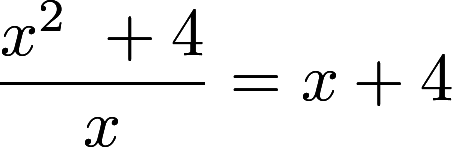• If you are citizen of an European Union member nation, you may not use this service unless you are at least 16 years old.

• Whenever you search in PBworks or on the Web, Dokkio Sidebar (from the makers of PBworks) will run the same search in your Drive, Dropbox, OneDrive, Gmail, Slack, and browsed web pages. Now you can find what you're looking for wherever it lives. Try Dokkio Sidebar for free.

View

# A2-F-2-1

last edited by 5 years, 2 months ago

A2.F.2.1 Add, subtract, multiply, and divide functions using function notation and recognize domain restrictions.

In a Nutshell

When functions are listed in function notation (i.e., f(x) or A(w)), operations can be applied to those functions similar to polynomial operations in most cases. These operations require reconsidering how the domain restrictions may have changed.

## Teacher Actions

• Students develop procedural fluency as they perform the four standard operations on polynomial rational and radical functions
• Implement mathematical tasks that provide students opportunities to explore how to use basic operations on functions, including various degrees of difficulty.

• Pose purposeful questions that allow students to discuss and explain when and why polynomials have or do not have a restriction of the domain.

• Elicit student thinking and evidence of understanding as the students analyze problems containing errors.

• Facilitate meaningful mathematical discourse by asking students  to reflect on work (their own and their classmates) and discuss common misconceptions.

• Develop procedural fluency by asking students to decompose polynomials using operations and given domain restrictions.

## Misconceptions

Procedural

• Perform basic operations on functions using order of operations, distributive, associative and commutative properties.

• Understand the names of the functions (f, g, h, Q, P or R) are not variables to be used as part of the arithmetic processes.

Conceptual

• Replace the notation of "y=" to the formal function notation f(x), g(x)...etc.

• Make a connection between basic operations on polynomial functions to the basic operations on numbers. Relate the process of performing basic operations on functions the same way as they are performed on polynomials

• Understand when and why the domain is restricted on a function.

• Understand how basic operations affect the graph of functions.

Identify and define each component of a function. (ie. Given: f(x) = -2x2 + 7 identify and define the following pieces: f, f(x), x, -2x2 + 7.

Common Mistakes and Related Errors

• Students misinterpret the notation f(x) to mean that f and x are being multiplied.

• Students fail to distribute subtraction to all parts of the subtrahend when it is a polynomial with more than one term.

• Students subtract or divide in the wrong order.

• Students only divide part of the numerator by the denominator.  For example:.

• Students fail to use the distributive property correctly.

• Students fail to combine like terms correctly.

• Students do not perform the multiplication of (a + b)n correctly [ex. Instead of (a + b)2 = a2 + 2ab + b2 they write (a + b)2 = a2 + b2]

Conceptual Issues

• Students fail to relate FOIL (memorized process) with distributive property. This could cause issues when multiplying a 3 term polynomial by a 2 term polynomial.

• Students fail to restrict the domain when dividing by a polynomial or taking the square root of a polynomial.

• Students fail to understand how basic operations affect the graph of polynomial functions.

OKMath Framework Introduction